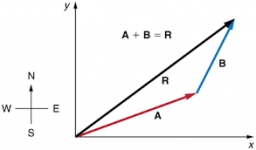# Direction 7999

A (5; -4) B (1; 3) C (-2; 0) D (6; 2)
Calculate the direction vector

a) a = AB
b) b = BC
c) c = CD

a0 =  -4
a1 =  7
b0 =  -3
b1 =  -3
c0 =  8
c1 =  2

### Step-by-step explanation:Did you find an error or inaccuracy? Feel free to write us. Thank you!

Tips for related online calculators
Our vector sum calculator can add two vectors given by their magnitudes and by included angle.